# Data for Titanic survival

Let’s see an example for DALEX package for classification models for the survival problem for Titanic dataset. Here we are using a dataset titanic avaliable in teh DALEX package. Note that this data was copied from the stablelearner package.

library("DALEX")
head(titanic)
#>   gender age class    embarked       country  fare sibsp parch survived
#> 1   male  42   3rd Southampton United States  7.11     0     0       no
#> 2   male  13   3rd Southampton United States 20.05     0     2       no
#> 3   male  16   3rd Southampton United States 20.05     1     1       no
#> 4 female  39   3rd Southampton       England 20.05     1     1      yes
#> 5 female  16   3rd Southampton        Norway  7.13     0     0      yes
#> 6   male  25   3rd Southampton United States  7.13     0     0      yes

# Model for Titanic survival

Ok, not it’s time to create a model. Let’s use the Random Forest model.

# prepare model
library("randomForest")
titanic <- na.omit(titanic)
model_titanic_rf <- randomForest(survived == "yes" ~ gender + age + class + embarked +
fare + sibsp + parch,  data = titanic)
model_titanic_rf
#>
#> Call:
#>  randomForest(formula = survived == "yes" ~ gender + age + class +      embarked + fare + sibsp + parch, data = titanic)
#>                Type of random forest: regression
#>                      Number of trees: 500
#> No. of variables tried at each split: 2
#>
#>           Mean of squared residuals: 0.1429882
#>                     % Var explained: 34.76

# Explainer for Titanic survival

The third step (it’s optional but useful) is to create a DALEX explainer for random forest model.

library("DALEX")
explain_titanic_rf <- explain(model_titanic_rf,
data = titanic[,-9],
y = titanic$survived == "yes", label = "Random Forest v7", colorize = FALSE) #> Preparation of a new explainer is initiated #> -> model label : Random Forest v7 #> -> data : 2099 rows 8 cols #> -> target variable : 2099 values #> -> predict function : yhat.randomForest will be used ( default ) #> -> predicted values : numerical, min = 0.007647857 , mean = 0.324321 , max = 0.9918327 #> -> residual function : difference between y and yhat ( default ) #> -> residuals : numerical, min = -0.782597 , mean = 0.0001191597 , max = 0.9035002 #> -> model_info : package randomForest , ver. 4.6.14 , task regression ( default ) #> A new explainer has been created! # Variable importance plots Use the variable_importance() explainer to present importance of particular features. Note that type = "difference" normalizes dropouts, and now they all start in 0. vi_rf <- variable_importance(explain_titanic_rf) head(vi_rf) #> variable mean_dropout_loss label #> 1 _full_model_ 112.8638 Random Forest v7 #> 2 country 112.8638 Random Forest v7 #> 3 parch 120.1363 Random Forest v7 #> 4 sibsp 121.1588 Random Forest v7 #> 5 embarked 124.6938 Random Forest v7 #> 6 fare 142.0032 Random Forest v7 plot(vi_rf)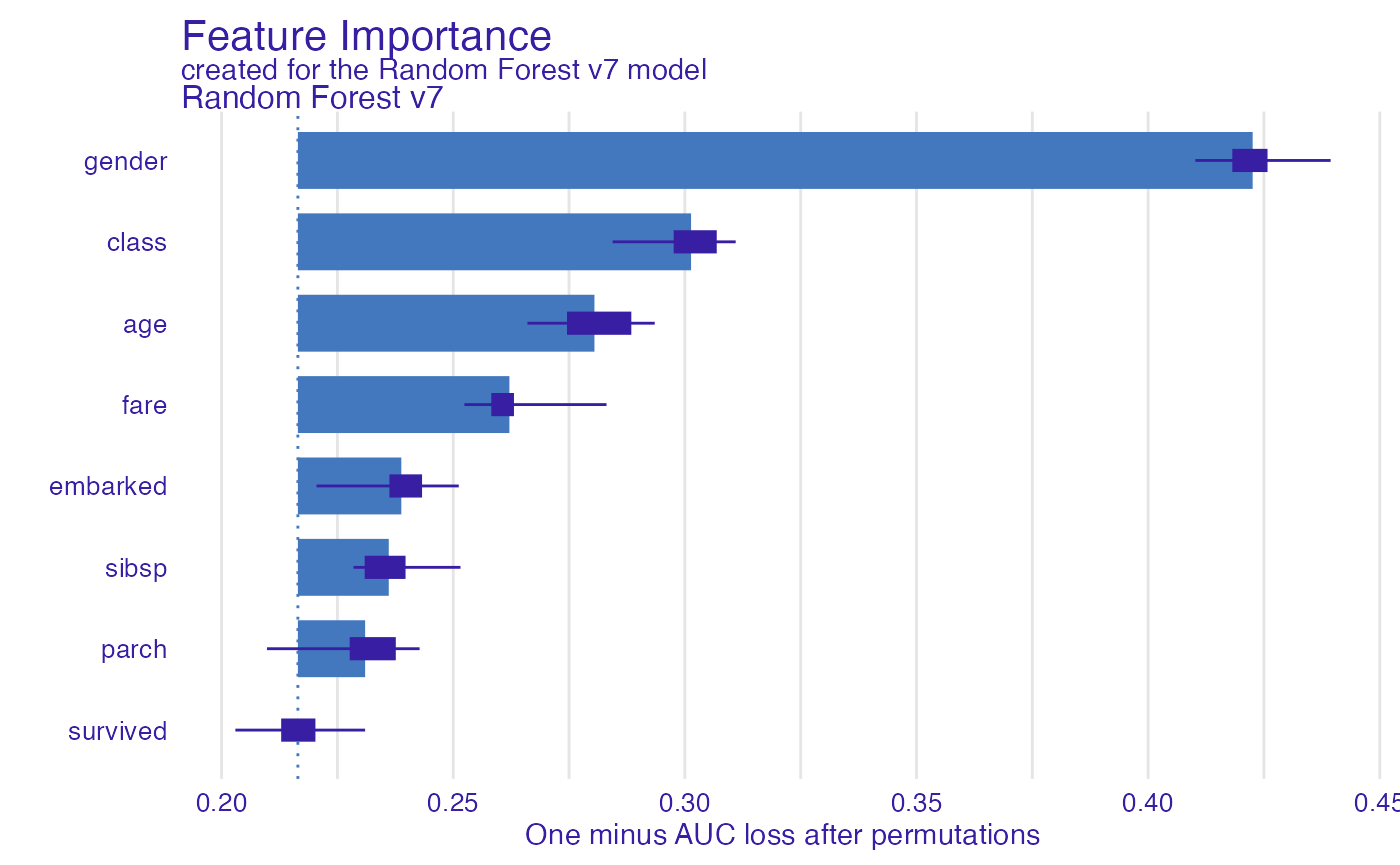# Variable effects As we see the most important feature is Sex. Next three importnat features are Pclass, Age and Fare. Let’s see the link between model response and these features. Such univariate relation can be calculated with variable_effect(). ## Age Kids 5 years old and younger have much higher survival probability. vr_age <- variable_effect(explain_titanic_rf, variables = "age") head(vr_age) #> Top profiles : #> _vname_ _label_ _x_ _yhat_ _ids_ #> 1 age Random Forest v7 0.1666667 0.5287876 0 #> 2 age Random Forest v7 2.0000000 0.5609795 0 #> 3 age Random Forest v7 4.0000000 0.5594695 0 #> 4 age Random Forest v7 7.0000000 0.5292187 0 #> 5 age Random Forest v7 9.0000000 0.5297403 0 #> 6 age Random Forest v7 13.0000000 0.4784188 0 plot(vr_age, use_facets = TRUE)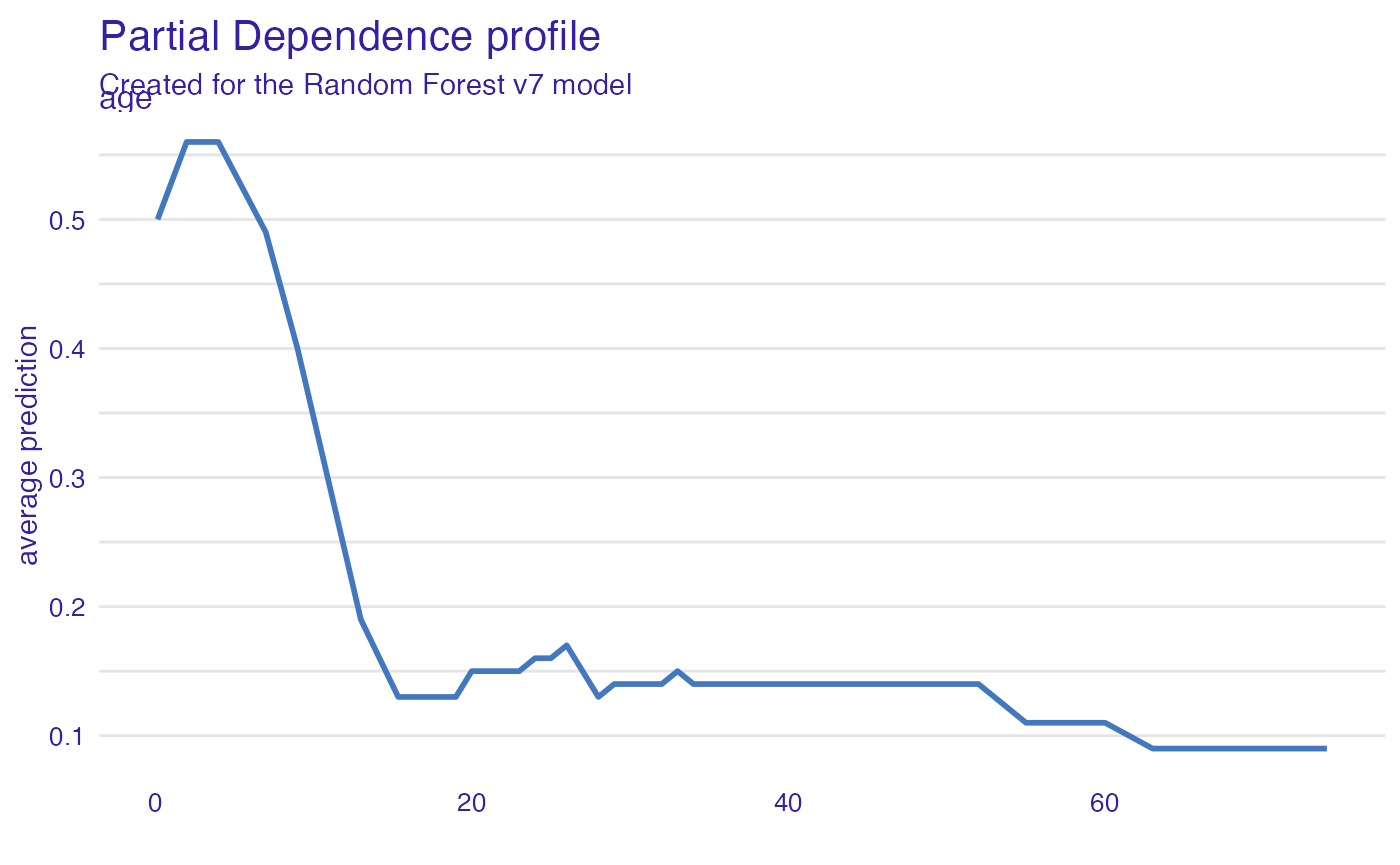## Passanger class Passangers in the first class have much higher survival probability. vr_class <- variable_effect(explain_titanic_rf, variables = "class") plot(vr_class)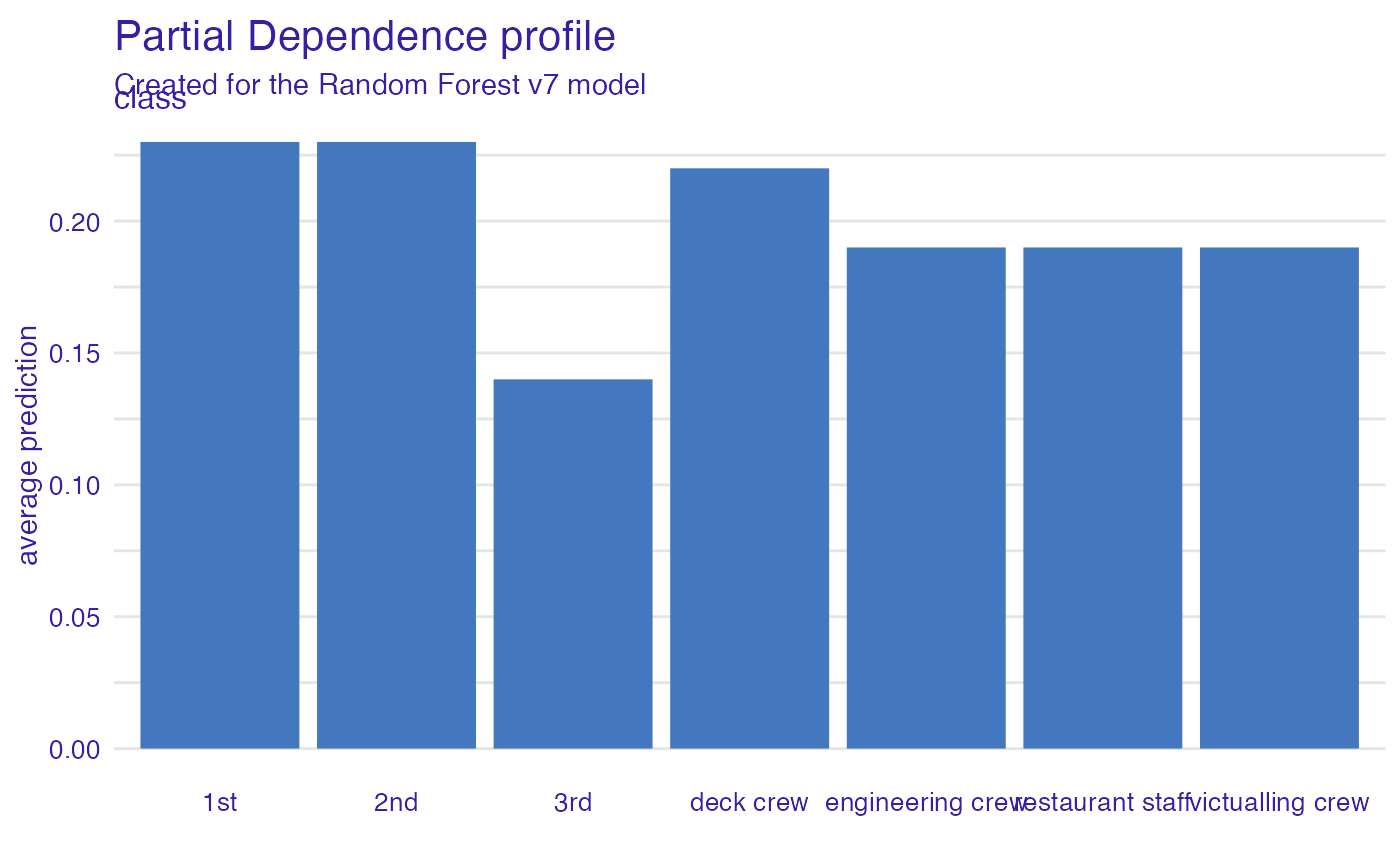## Fare Very cheap tickets are linked with lower chances. vr_fare <- variable_effect(explain_titanic_rf, variables = "fare") plot(vr_fare, use_facets = TRUE)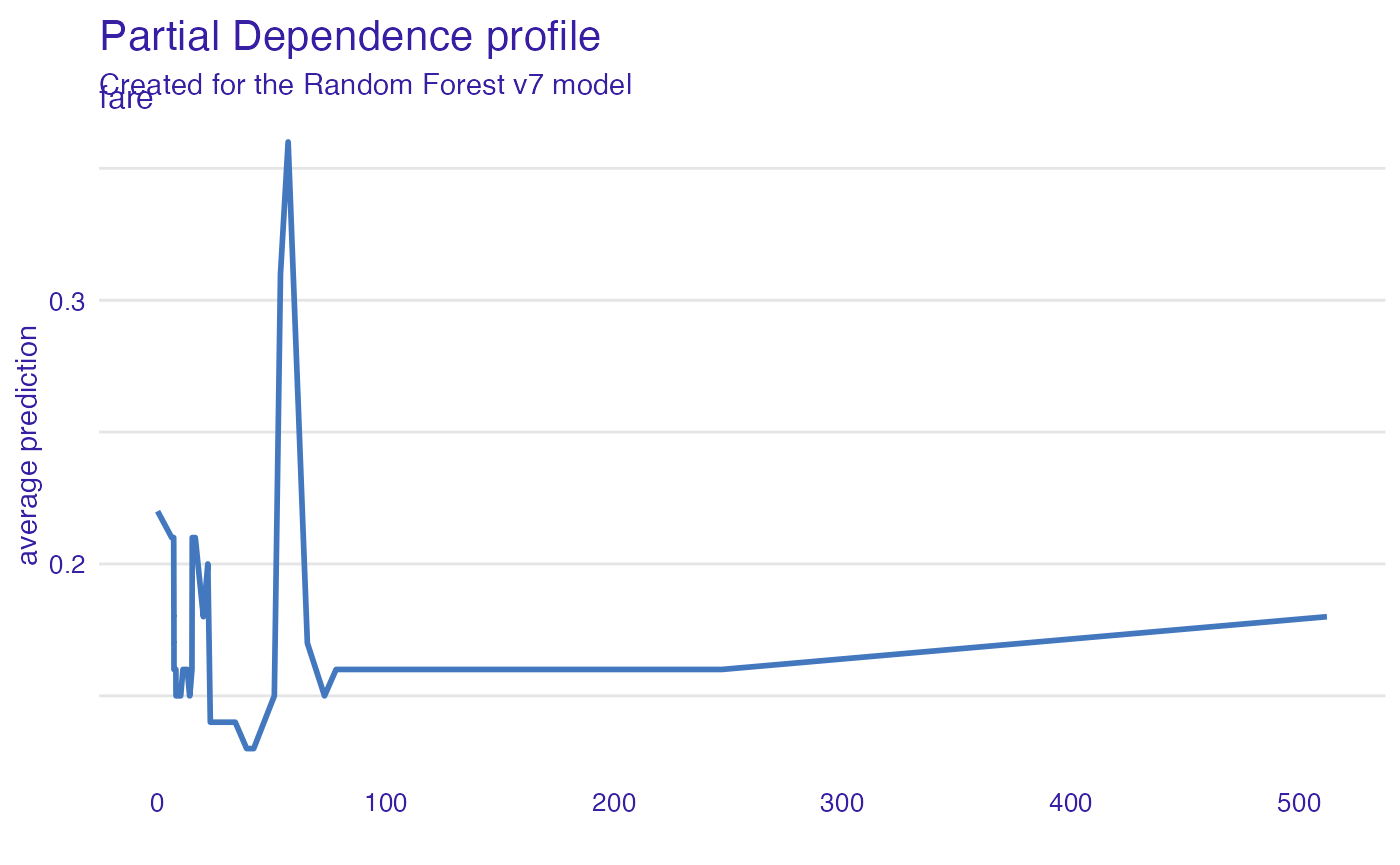## Embarked Passangers that embarked from C have highest survival. vr_embarked <- variable_effect(explain_titanic_rf, variables = "embarked") plot(vr_embarked)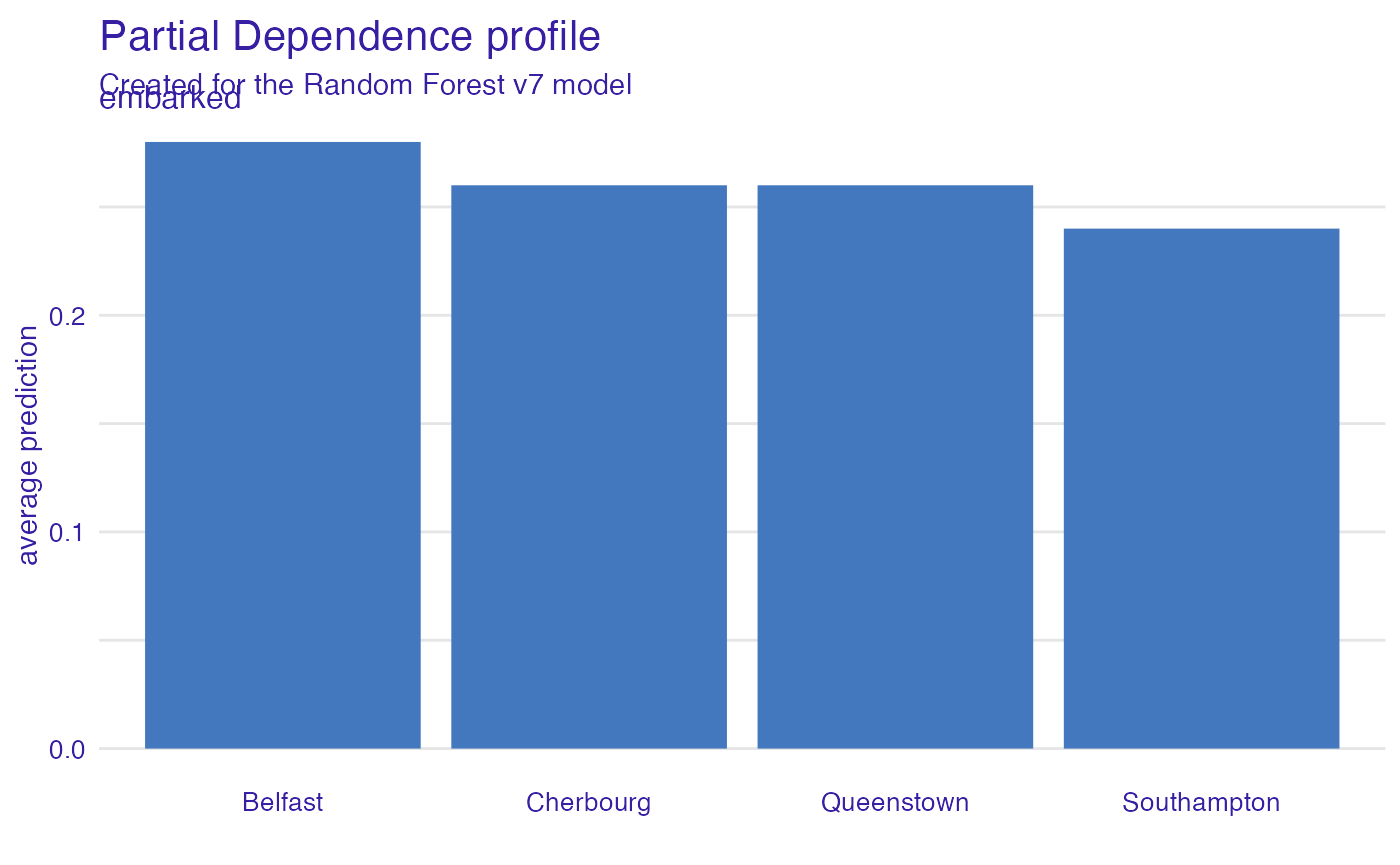# Instance level explanations Let’s see break down explanation for model predictions for 8 years old male from 1st class that embarked from port C. new_passanger <- data.frame( class = factor("1st", levels = c("1st", "2nd", "3rd", "deck crew", "engineering crew", "restaurant staff", "victualling crew")), gender = factor("male", levels = c("female", "male")), age = 8, sibsp = 0, parch = 0, fare = 72, embarked = factor("Southampton", levels = c("Belfast", "Cherbourg", "Queenstown", "Southampton")) ) sp_rf <- variable_attribution(explain_titanic_rf, new_passanger) plot(sp_rf)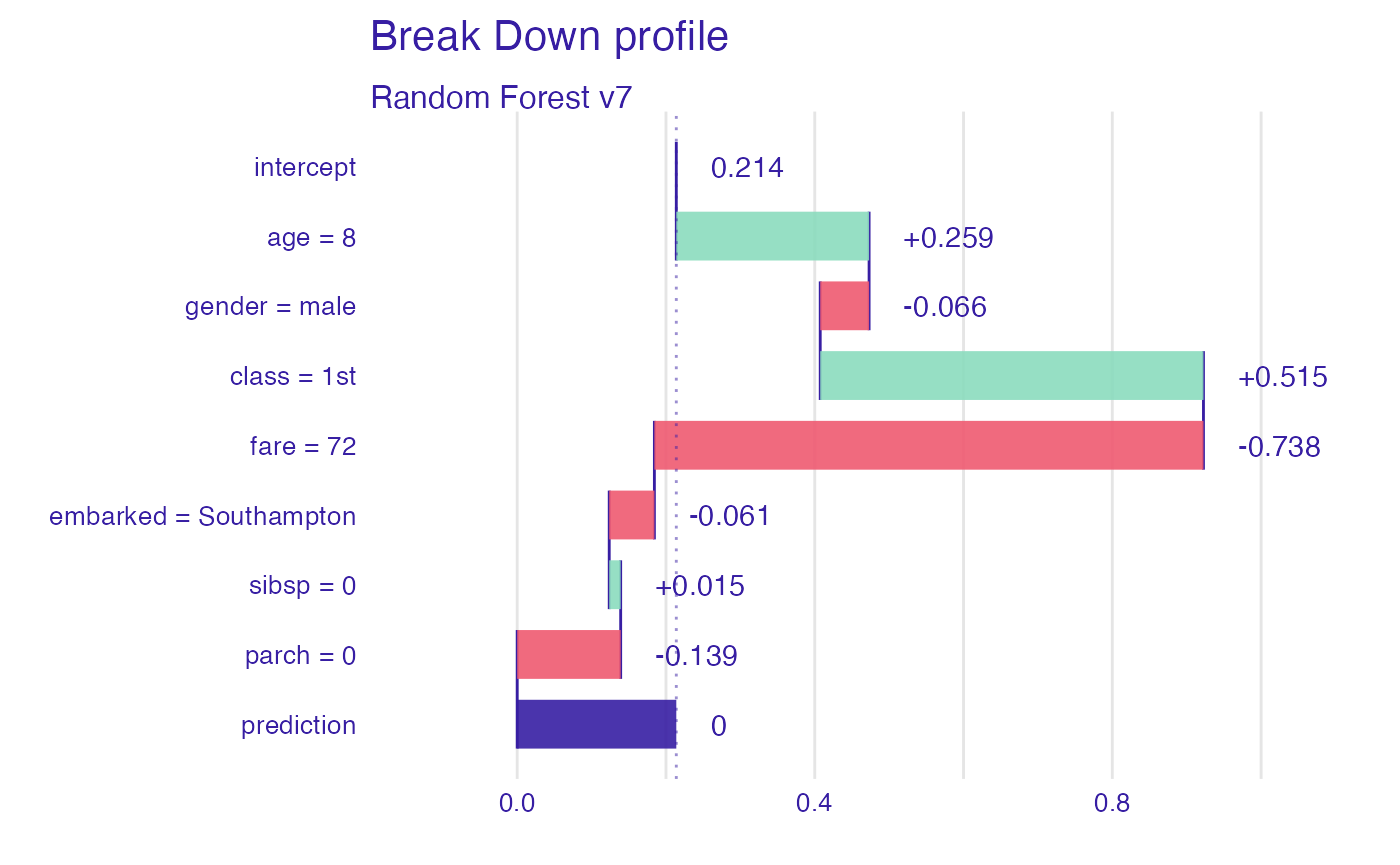It looks like the most important feature for this passenger is age and sex. After all his odds for survival are higher than for the average passenger. Mainly because of the young age and despite of being a male. # More models Let’s train more models for survival. ## Logistic regression library("rms") model_titanic_lmr <- lrm(survived == "yes" ~ class + gender + rcs(age) + sibsp + parch + fare + embarked, titanic) explain_titanic_lmr <- explain(model_titanic_lmr, data = titanic, y = titanic$survived == "yes",
predict_function = function(m,x) predict(m, x, type="fitted"),
label = "Logistic regression")
#> Preparation of a new explainer is initiated
#>   -> model label       :  Logistic regression
#>   -> data              :  2099  rows  9  cols
#>   -> target variable   :  2099  values
#>   -> predict function  :  function(m, x) predict(m, x, type = "fitted")
#>   -> predicted values  :  numerical, min =  0.003695743 , mean =  0.3244402 , max =  0.9827164
#>   -> residual function :  difference between y and yhat ( [33m default [39m )
#>   -> residuals         :  numerical, min =  -0.9827164 , mean =  -2.869171e-09 , max =  0.9716889
#>   -> model_info        :  package stats , ver. 3.6.1 , task regression ( [33m default [39m )
#>  [32m A new explainer has been created! [39m

## Generalized Boosted Models (GBM)

library("gbm")
model_titanic_gbm <- gbm(survived == "yes" ~ class + gender + age + sibsp +
parch + fare + embarked, data = titanic, n.trees = 15000)
#> Distribution not specified, assuming bernoulli ...
explain_titanic_gbm <- explain(model_titanic_gbm, data = titanic,
y = titanic$survived == "yes", predict_function = function(m,x) predict(m, x, n.trees = 15000, type = "response"), label = "Generalized Boosted Models", colorize = FALSE) #> Preparation of a new explainer is initiated #> -> model label : Generalized Boosted Models #> -> data : 2099 rows 9 cols #> -> target variable : 2099 values #> -> predict function : function(m, x) predict(m, x, n.trees = 15000, type = "response") #> -> predicted values : numerical, min = 0.0001458708 , mean = 0.322447 , max = 0.9984808 #> -> residual function : difference between y and yhat ( default ) #> -> residuals : numerical, min = -0.9384621 , mean = 0.001993254 , max = 0.9589537 #> -> model_info : package gbm , ver. 2.1.5 , task classification ( default ) #> A new explainer has been created! ## Support Vector Machines (SVM) library("e1071") model_titanic_svm <- svm(survived == "yes" ~ class + gender + age + sibsp + parch + fare + embarked, data = titanic, type = "C-classification", probability = TRUE) explain_titanic_svm <- explain(model_titanic_svm, data = titanic, y = titanic$survived == "yes",
label = "Support Vector Machines",
colorize = FALSE)
#> Preparation of a new explainer is initiated
#>   -> model label       :  Support Vector Machines
#>   -> data              :  2099  rows  9  cols
#>   -> target variable   :  2099  values
#>   -> predict function  :  yhat.svm  will be used (  default  )
#>   -> predicted values  :  numerical, min =  0.08638015 , mean =  0.3277075 , max =  0.9581973
#>   -> residual function :  difference between y and yhat (  default  )
#>   -> residuals         :  numerical, min =  -0.8565142 , mean =  -0.00326728 , max =  0.8904463
#>   -> model_info        :  package e1071 , ver. 1.7.2 , task classification (  default  )
#>   A new explainer has been created!

## k-Nearest Neighbours (kNN)

library("caret")
model_titanic_knn <- knn3(survived == "yes" ~ class + gender + age + sibsp +
parch + fare + embarked, data = titanic, k = 5)
explain_titanic_knn <- explain(model_titanic_knn, data = titanic,
y = titanic\$survived == "yes",
predict_function = function(m,x) predict(m, x)[,2],
label = "k-Nearest Neighbours",
colorize = FALSE)
#> Preparation of a new explainer is initiated
#>   -> model label       :  k-Nearest Neighbours
#>   -> data              :  2099  rows  9  cols
#>   -> target variable   :  2099  values
#>   -> predict function  :  function(m, x) predict(m, x)[, 2]
#>   -> predicted values  :  numerical, min =  0 , mean =  0.3110276 , max =  1
#>   -> residual function :  difference between y and yhat (  default  )
#>   -> residuals         :  numerical, min =  -0.8 , mean =  0.01341259 , max =  0.9285714
#>   -> model_info        :  package Model of class: knn3 package unrecognized , ver. Unknown , task regression (  default  )
#>   A new explainer has been created!

## Variable performance

vi_rf <- variable_importance(explain_titanic_rf)
vi_lmr <- variable_importance(explain_titanic_lmr)
vi_gbm <- variable_importance(explain_titanic_gbm)
vi_svm <- variable_importance(explain_titanic_svm)
vi_knn <- variable_importance(explain_titanic_knn)

plot(vi_rf, vi_lmr, vi_gbm, vi_svm, vi_knn, bar_width = 4)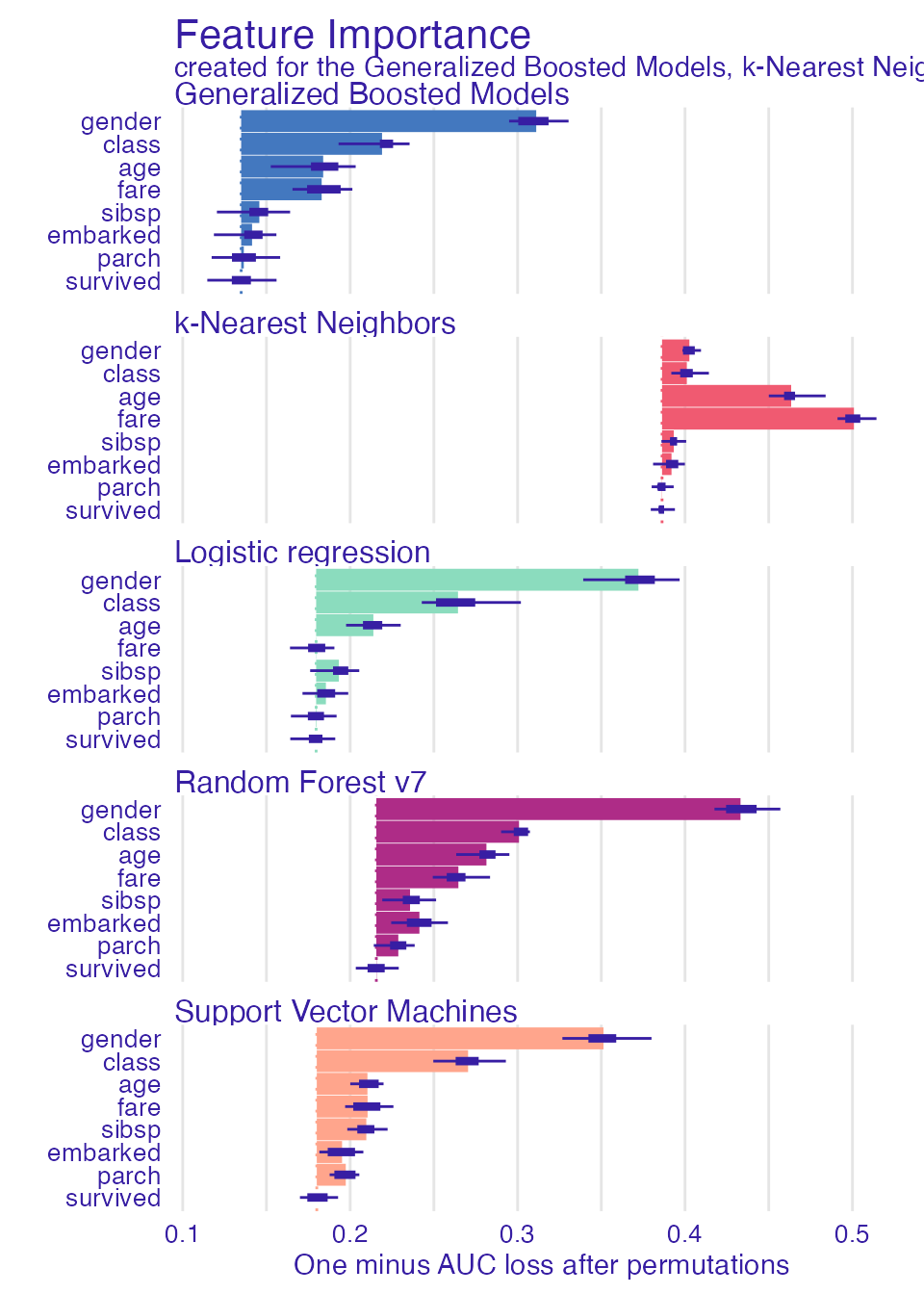## Single variable

vr_age_rf  <- variable_effect(explain_titanic_rf, variables =  "age")
vr_age_lmr  <- variable_effect(explain_titanic_lmr, variables =  "age")
vr_age_gbm  <- variable_effect(explain_titanic_gbm, variables =  "age")
vr_age_svm  <- variable_effect(explain_titanic_svm, variables =  "age")
vr_age_knn  <- variable_effect(explain_titanic_knn, variables =  "age")
plot(vr_age_rf, vr_age_lmr, vr_age_gbm, vr_age_svm, vr_age_knn)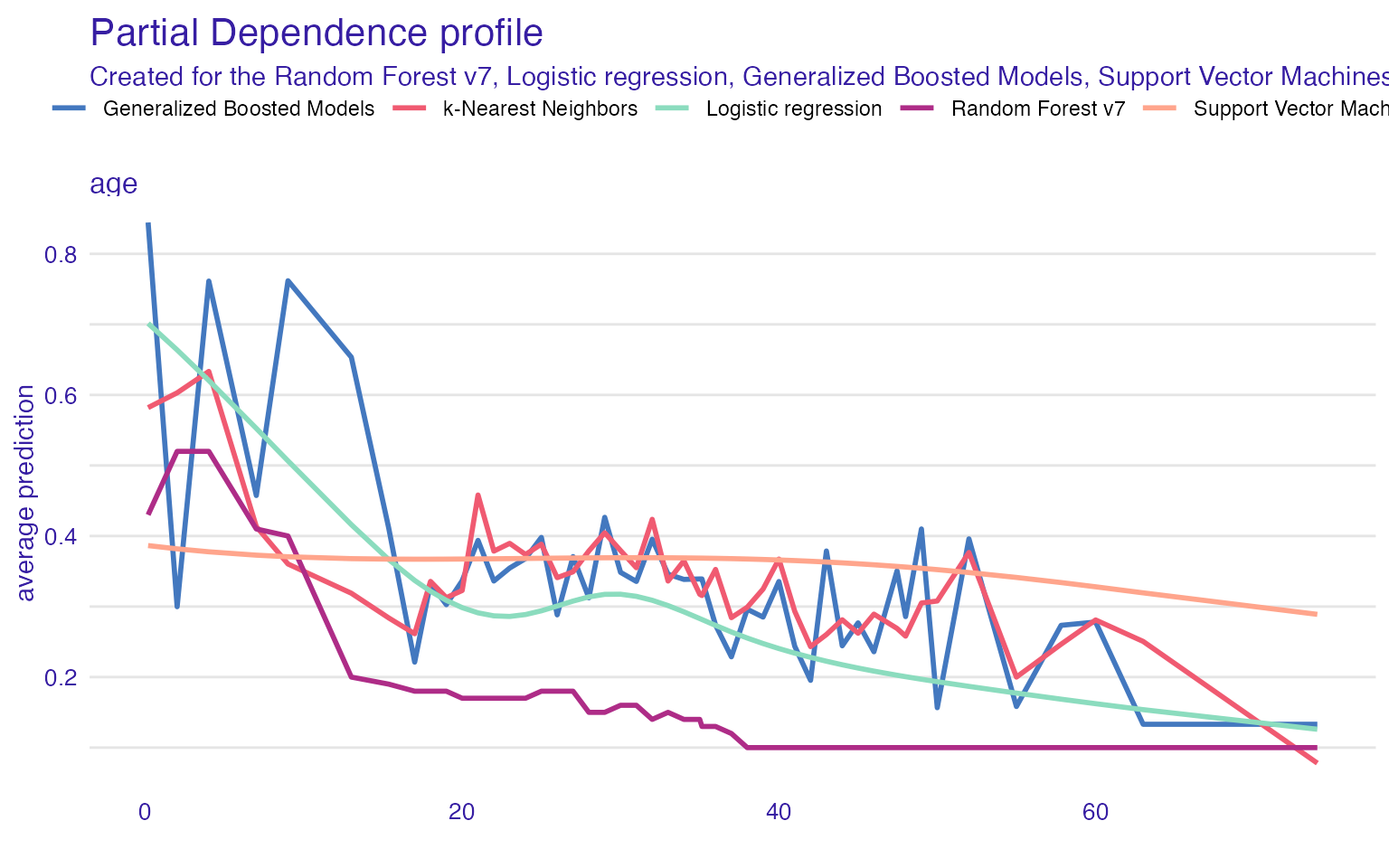plot(vr_age_rf, vr_age_lmr, vr_age_gbm, vr_age_svm, vr_age_knn, use_facets = TRUE)# Instance level explanations

sp_rf <- variable_attribution(explain_titanic_rf, new_passanger)
plot(sp_rf, sp_lmr, sp_gbm, sp_svm, sp_knn)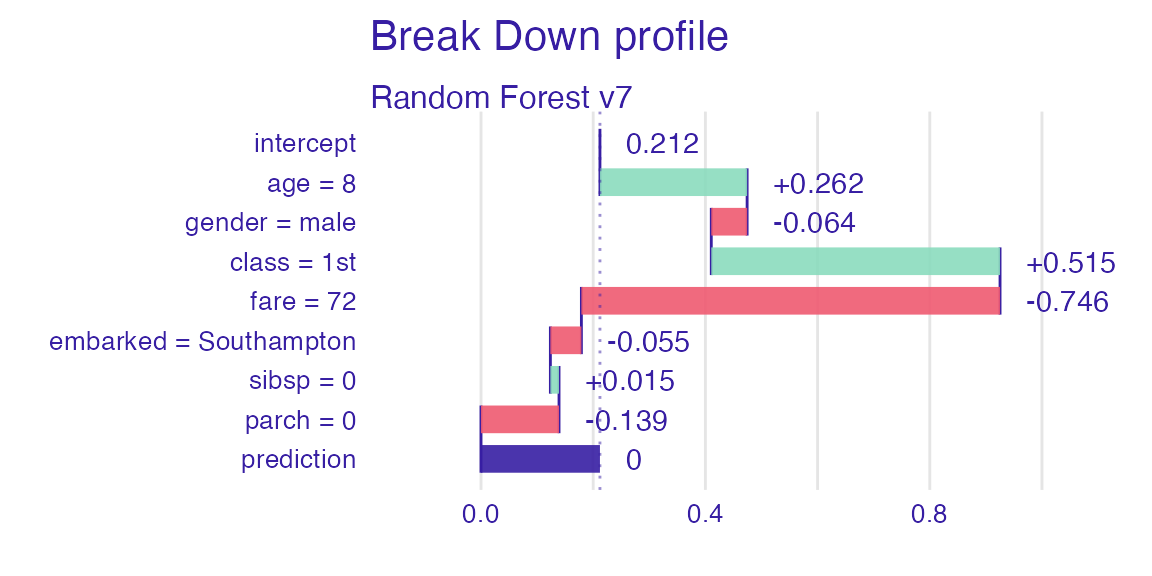# Session info

sessionInfo()
#> R version 3.6.1 (2019-07-05)
#> Platform: x86_64-apple-darwin15.6.0 (64-bit)
#> Running under: macOS Mojave 10.14.4
#>
#> Matrix products: default
#> BLAS:   /Library/Frameworks/R.framework/Versions/3.6/Resources/lib/libRblas.0.dylib
#> LAPACK: /Library/Frameworks/R.framework/Versions/3.6/Resources/lib/libRlapack.dylib
#>
#> locale:
#>  en_US.UTF-8/en_US.UTF-8/en_US.UTF-8/C/en_US.UTF-8/en_US.UTF-8
#>
#> attached base packages:
#>  stats     graphics  grDevices utils     datasets  methods   base
#>
#> other attached packages:
#>   caret_6.0-84        e1071_1.7-2         gbm_2.1.5
#>   rms_5.1-3.1         SparseM_1.77        Hmisc_4.2-0
#>   ggplot2_3.2.1       Formula_1.2-3       survival_2.44-1.1
#>  lattice_0.20-38     randomForest_4.6-14 DALEX_0.9.1
#>
#> loaded via a namespace (and not attached):
#>   foreach_1.4.7       splines_3.6.1       prodlim_2018.04.18
#>   assertthat_0.2.1    stats4_3.6.1        ingredients_0.5.1
#>   latticeExtra_0.6-28 yaml_2.2.0          ipred_0.9-9
#>  pillar_1.4.2        backports_1.1.5     quantreg_5.51
#>  glue_1.3.1          digest_0.6.23       RColorBrewer_1.1-2
#>  checkmate_1.9.4     recipes_0.1.7       colorspace_1.4-1
#>  sandwich_2.5-1      plyr_1.8.4          htmltools_0.4.0
#>  Matrix_1.2-17       timeDate_3043.102   pkgconfig_2.0.3
#>  purrr_0.3.3         mvtnorm_1.0-11      scales_1.1.0
#>  gower_0.2.1         lava_1.6.6          MatrixModels_0.4-1
#>  htmlTable_1.13.2    tibble_2.1.3        generics_0.0.2
#>  farver_2.0.1        TH.data_1.0-10      withr_2.1.2
#>  nnet_7.3-12         lazyeval_0.2.2      magrittr_1.5
#>  crayon_1.3.4        memoise_1.1.0       polspline_1.1.16
#>  evaluate_0.14       fs_1.3.1            nlme_3.1-140
#>  MASS_7.3-51.4       foreign_0.8-71      class_7.3-15
#>  tools_3.6.1         data.table_1.12.6   lifecycle_0.1.0
#>  multcomp_1.4-10     stringr_1.4.0       munsell_0.5.0
#>  cluster_2.1.0       compiler_3.6.1      pkgdown_1.4.1
#>  rlang_0.4.2         grid_3.6.1          iterators_1.0.12
#>  rstudioapi_0.10     htmlwidgets_1.5.1   base64enc_0.1-3
#>  labeling_0.3        rmarkdown_1.16      ModelMetrics_1.2.2
#>  gtable_0.3.0        codetools_0.2-16    reshape2_1.4.3
#>  R6_2.4.1            lubridate_1.7.4     gridExtra_2.3
#>  zoo_1.8-6           knitr_1.26          dplyr_0.8.3
#>  rprojroot_1.3-2     iBreakDown_0.9.9    desc_1.2.0
#>  stringi_1.4.3       Rcpp_1.0.3          rpart_4.1-15
#>  acepack_1.4.1       tidyselect_0.2.5    xfun_0.11